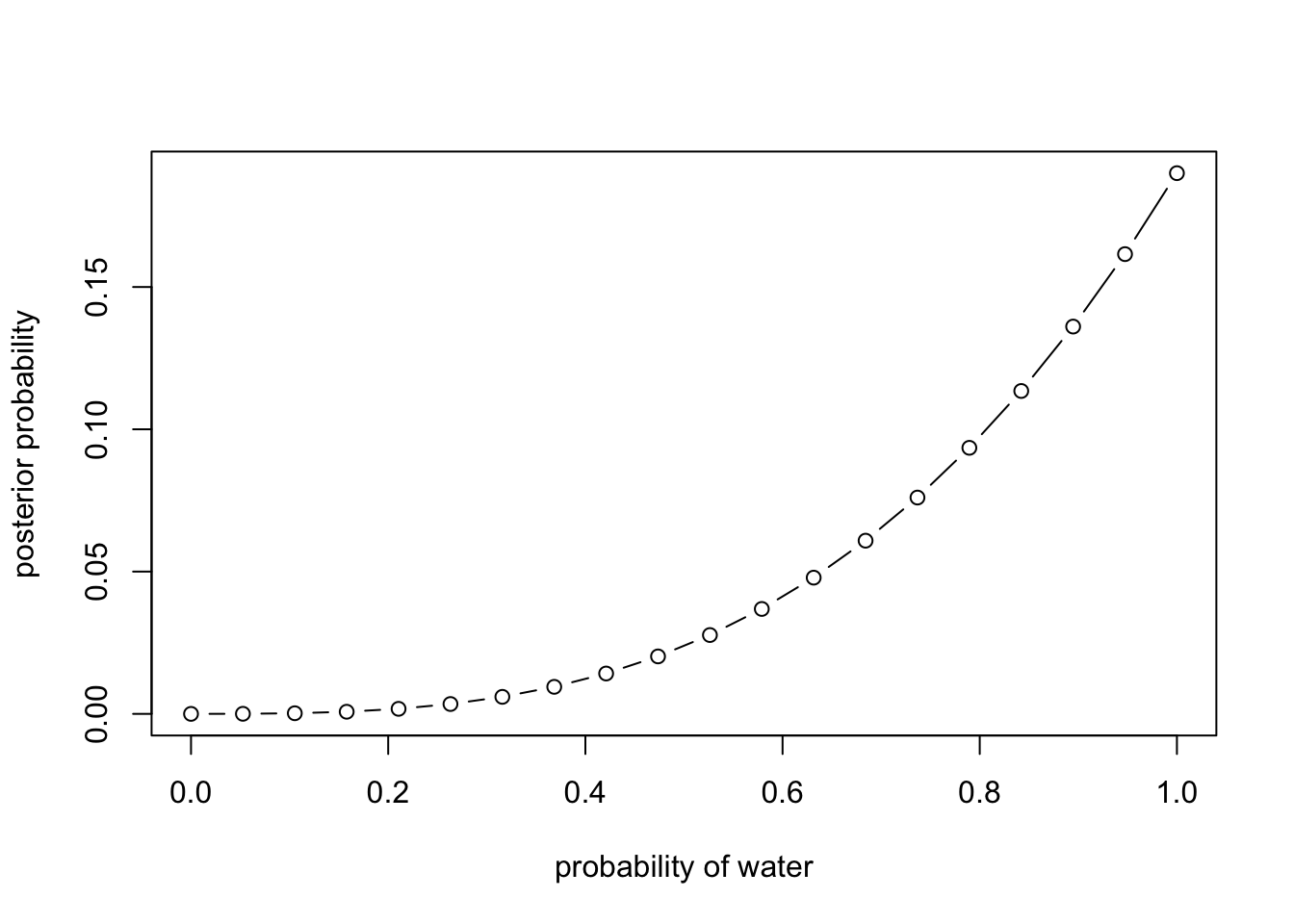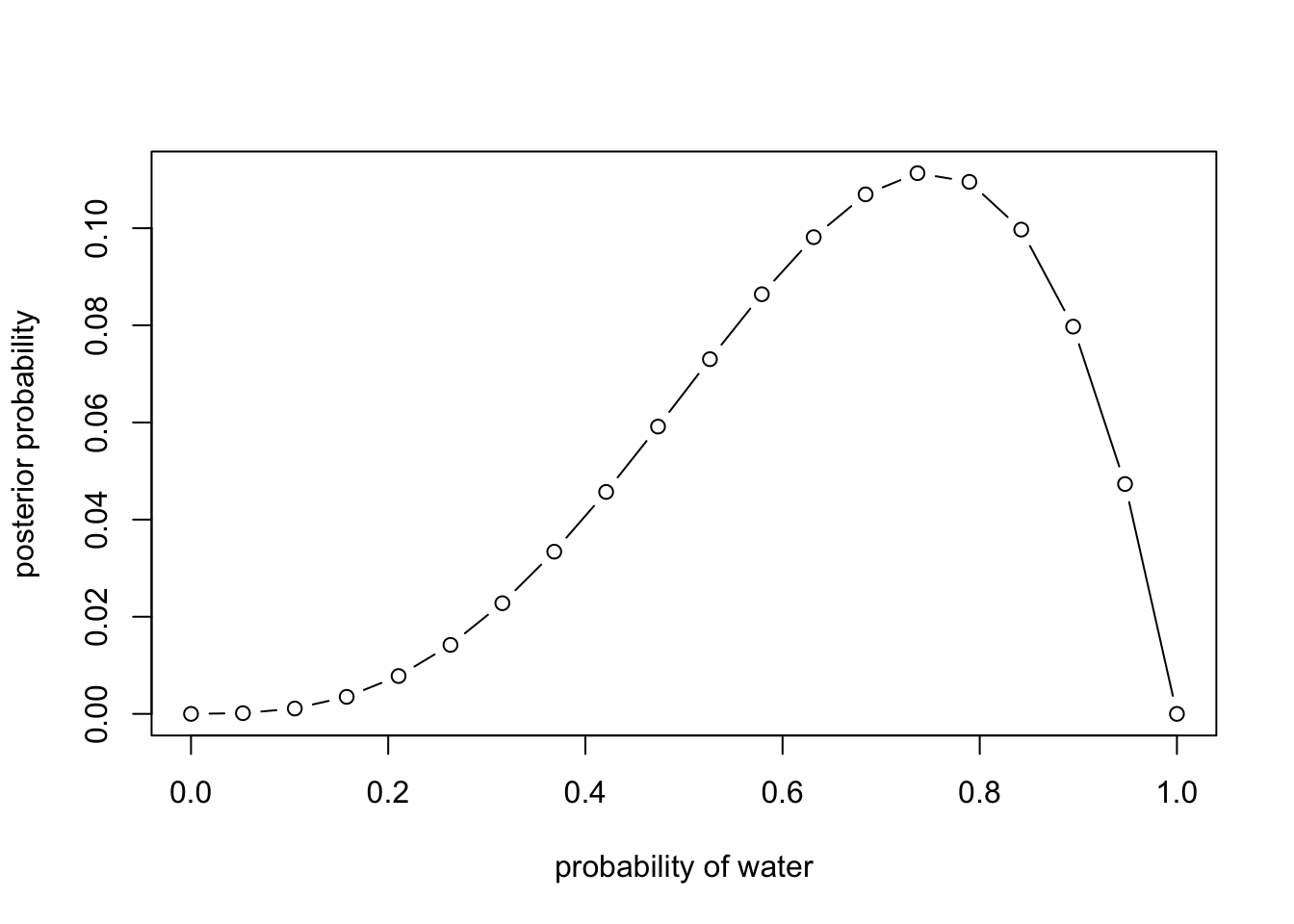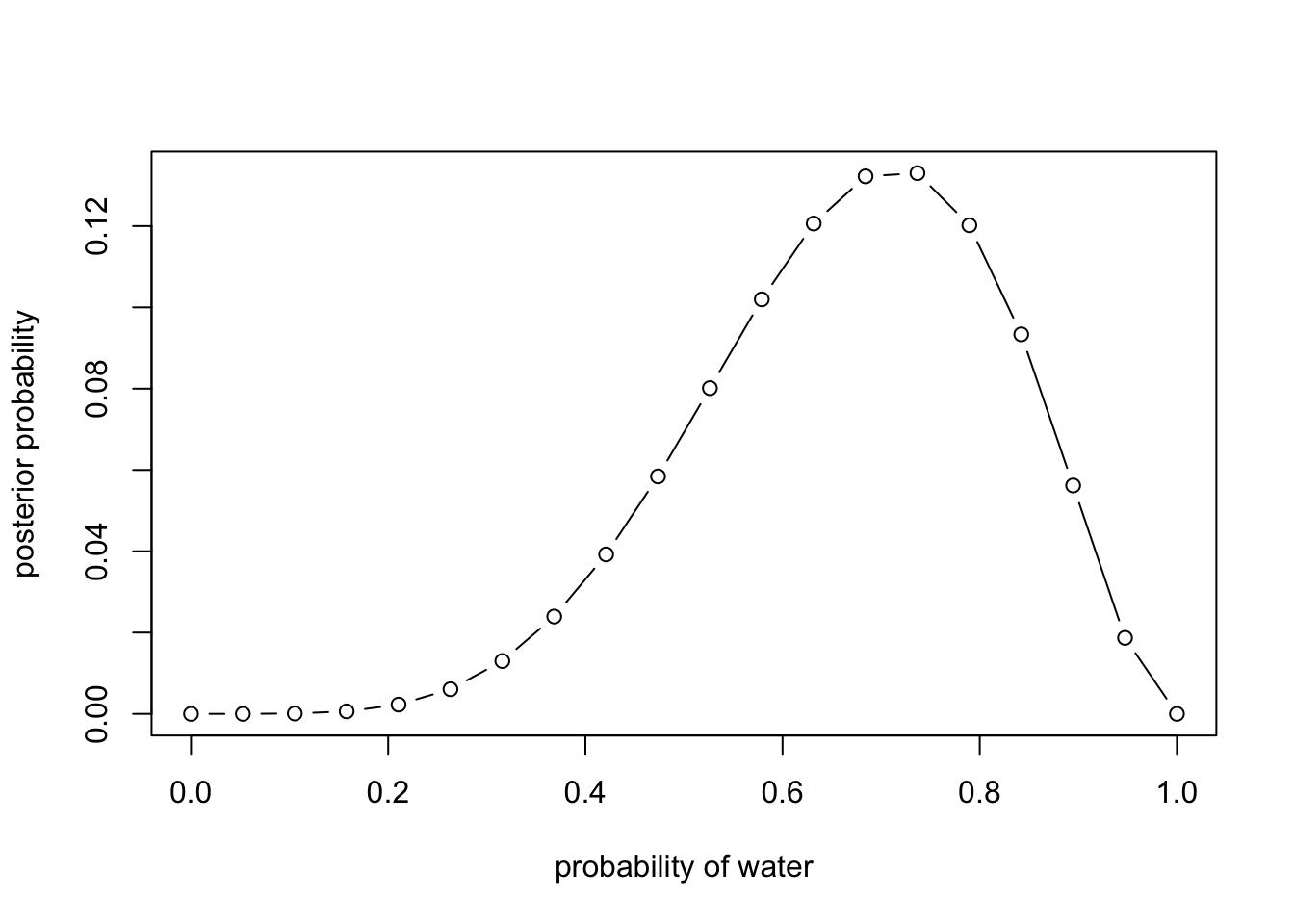## Chapter 2 - Large Worlds and Small Worlds

The objectives of this problem set is to work with the conceptual mechanics of Bayesian data analysis. The target of inference in Bayesian inference is a posterior probability distribution. Posterior probabilities state the relative numbers of ways each conjectured cause of the data could have produced the data. These relative numbers indicate plausibilities of the different conjectures. These plausibilities are updated in light of observations through Bayesian updating.

Place each answer inside the code chunk (grey box). The code chunks should contain a text response or a code that completes/answers the question or activity requested. Make sure to include plots if the question requests them. Problems are labeled Easy (E), Medium (M), and Hard(H).

Finally, upon completion, name your final output `.html` file as: `YourName_ANLY505-Year-Semester.html` and publish the assignment to your R Pubs account and submit the link to Canvas. Each question is worth 5 points

## Questions

2E1. Which of the expressions below correspond to the statement: the probability of rain on Monday? (1) Pr(rain) (2) Pr(rain|Monday) (3) Pr(Monday|rain) (4) Pr(rain, Monday)/ Pr(Monday)

``#(2) and (4) are correct.``

2E2. Which of the following statements corresponds to the expression: Pr(Monday|rain)? (1) The probability of rain on Monday. (2) The probability of rain, given that it is Monday. (3) The probability that it is Monday, given that it is raining. (4) The probability that it is Monday and that it is raining.

``#(3) is correct.``

2E3. Which of the expressions below correspond to the statement: the probability that it is Monday, given that it is raining? (1) Pr(Monday|rain) (2) Pr(rain|Monday) (3) Pr(rain|Monday) Pr(Monday) (4) Pr(rain|Monday) Pr(Monday)/ Pr(rain) (5) Pr(Monday|rain) Pr(rain)/ Pr(Monday)

``#(1) and (4) are correct.``

2E4. The Bayesian statistician Bruno de Finetti (1906–1985) began his 1973 book on probability theory with the declaration: “PROBABILITY DOES NOT EXIST.” The capitals appeared in the original, so I imagine de Finetti wanted us to shout this statement. What he meant is that probability is a device for describing uncertainty from the perspective of an observer with limited knowledge; it has no objective reality. Discuss the globe tossing example from the chapter, in light of this statement. What does it mean to say “the probability of water is 0.7”?

``#It means that from the perspective of an observer with limited knowledge, the estimate value of this parameter - water is 0.7.``

2M1. Recall the globe tossing model from the chapter. Compute and plot the grid approximate posterior distribution for each of the following sets of observations. In each case, assume a uniform prior for p. (1) W, W, W (2) W, W, W, L (3) L, W, W, L, W, W, W

``````#(1)
p_grid <- seq(from = 0, to = 1, length.out = 20)
prior <- rep(1, 20)
likelihood <- dbinom(3, size = 3, prob = p_grid)
unstd.posterior <- likelihood * prior
posterior <- unstd.posterior / sum(unstd.posterior)
plot(p_grid, posterior, type = "b",
xlab = "probability of water", ylab = "posterior probability")````````````#(2)
p_grid <- seq(from = 0, to = 1, length.out = 20)
prior <- rep(1, 20)
likelihood <- dbinom(3, size = 4, prob = p_grid)
unstd.posterior <- likelihood * prior
posterior <- unstd.posterior / sum(unstd.posterior)
plot(p_grid, posterior, type = "b",
xlab = "probability of water", ylab = "posterior probability")````````````#(3)
p_grid <- seq(from = 0, to = 1, length.out = 20)
prior <- rep(1, 20)
likelihood <- dbinom(5, size = 7, prob = p_grid)
unstd.posterior <- likelihood * prior
posterior <- unstd.posterior / sum(unstd.posterior)
plot(p_grid, posterior, type = "b",
xlab = "probability of water", ylab = "posterior probability")``````2M2. Now assume a prior for p that is equal to zero when p < 0.5 and is a positive constant when p ≥ 0.5. Again compute and plot the grid approximate posterior distribution for each of the sets of observations in the problem just above.

``````p_grid <- seq(from = 0, to = 1, length.out = 20)
prior <- ifelse(p_grid < 0.5, 0, 1)
likelihood <- dbinom(3, size = 3, prob = p_grid)
unstd.posterior <- likelihood * prior
posterior <- unstd.posterior / sum(unstd.posterior)
plot(p_grid, posterior, type = "b",
xlab = "probability of water", ylab = "posterior probability")``````